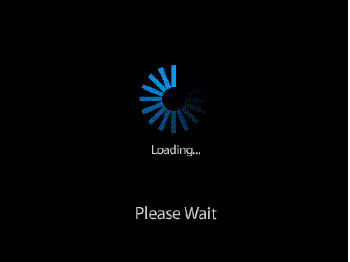APPOr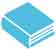OG20官方指南，建议同学们刷3遍。第一遍做题了解题型和考点，第二遍精刷，第三遍集中解决疑难问题。建议考生第一遍刷题采用官方正版纸质书籍，若遇到疑难问题，欢迎在此专区查阅解析，提供解析，参与题目讨论，与所有考生一起解决疑难问题。

##### 请描述一下这个错误：

【OG20-P286-380题】

If the positive integer n is added to each of the integers 69, 94, and 121,what is the value of n ?

(1) 69+ n and 94 + n are the squares of two consecutive integers.

(2) 94 + n and 121 + n are the squares of two consecutive integers.

• 分析A选项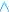xxxxxxxxxxxxxxxxxxxxxxxxxxxxxxxxxxxx
• 分析B选项xxxxxxxxxxxxxxxxxxxxxxxxxxxxxxxxxxxx
• 分析C选项xxxxxxxxxxxxxxxxxxxxxxxxxxxxxxxxxxxx
• 分析D选项xxxxxxxxxxxxxxxxxxxxxxxxxxxxxxxxxxxx
• 分析E选项xxxxxxxxxxxxxxxxxxxxxxxxxxxxxxxxxxxx暂无雷哥网文字解析

`条件1:69+n=x^2,94+n=(x+1)^2解得x=12,n=75，充分条件2:94+n=y^2,121+n=(y+1)^2解得y=13,n=75，充分`

### 题目讨论 （3条评论）•kikig

```From statement one, we can say that for some positive integer x, 69 + n = x^2 and 94 + n = (x + 1)^2. Let’s subtract the first equation from the second equation:

(94 + n) - (69 + n) = (x + 1)^2 - x^2```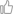0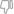0 回复 2019-12-18 16:58:49
•phylliskong38

`2 unknown 2 equations ---> solvable`00 回复 2018-08-28 22:46:00
•```1)69+n=a^2
94+n=(a+1)^2
上下相减，a=12, n=75   充分

2) 同样的方法  94+n=b^2
121+n=(b+1)^2
上下相减， b=13, n=75  充分```00 回复 2018-02-25 21:53:03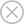• 手机注册
• 邮箱注册
登录＞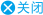###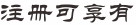•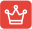#### 知识库学习

GMAT语法、逻辑、阅读、数学各单项备考知识点学习及测验
•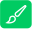#### 在线做题

包含GMAT各单项必考知识点题目、OG/PREP/GWD/雷哥讲义题目、难度650/680/700/730题库题目练习及题目解析
•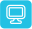#### 在线模考

语文套题/数学套题/全套仿真模考，包含GWD/PERP/精选模考等上百套套题模考
•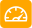#### 在线测评

适合5种不同基础的GMAT学员，测评后可自动出具分数报告及复习计划指导
•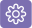#### 资料下载

GMAT必备备考资料下载、鸡精下载、课程课件等免费下载
•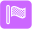#### 课程学习

注册会员后，可在GMAT课程区，选择免费直播课程及公开课程进行在线学习
×
请你选择你要查看的模考成绩单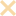立即开通 暂不开通